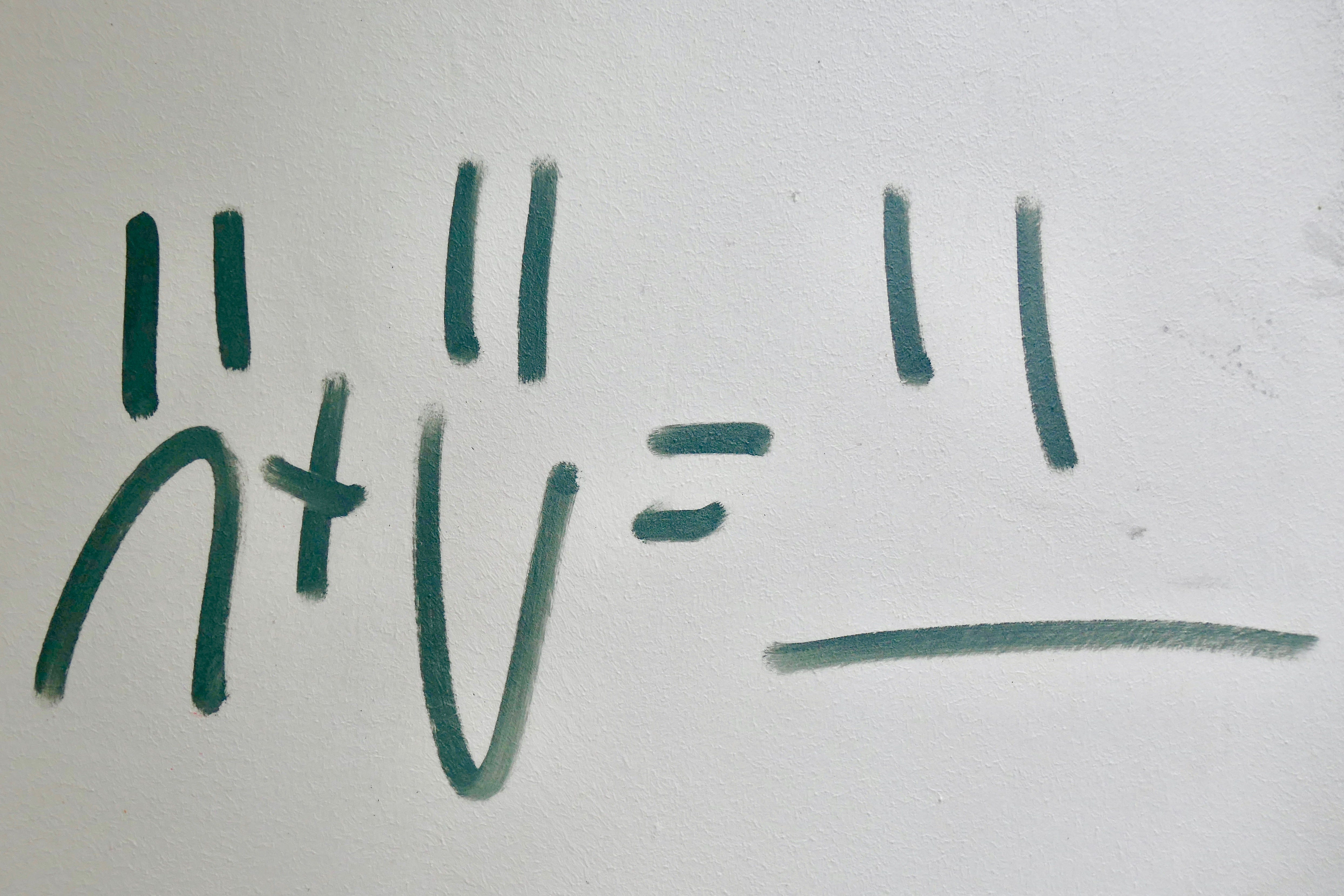# Mathematical Notation for Python Developers | Propositional Logic## Introduction

As developers, we require to be always up to date with the new tech stack however we tend to forget the importance of the underlying structure in which new tech stacks are built-in.

I am not saying we are required to be ninjas at assembly or binary but a good understanding of how they work is necessary in order to progress as a developer.

Learning Propositional Logic can help you understand how circuits work programming conditions, writing your code with mathematical notation amongst other things.By now, you might be wondering: “Do I need to know Python or Binary to understand this article?”. You do not!

## Propositional Logic

### AND | Conjunction

AND expressions are denoted with the symbol `∧`.

a ∧ b = a and b

Both a and b must be true in order for an AND statement to be true or else it will always be false.

|1 ∧ 0 = 0|0 ∧ 1 = 0|1 ∧ 1 = 1 |0 ∧ 0 = 0|

### OR | Disjunction

OR expressions are denoted with the symbol `∨`.

a ∨ b = a or b

Either a or b must be true in order for an OR statement to be true.

|1 ∨ 0 = 1|0 ∨ 1 = 1|1 ∨ 1 = 1|0 ∨ 0 = 0 |

### NOT | Negation

NOT expressions are denoted with the symbols `¬` or `~`.

If a = 1 then ~a = 0 or vice versa

Hold on tight it is about to get a bit more complex trust me we can do this together.

### Exclusive OR | Exclusive Disjunction | XOR

Exclusive OR expressions are denoted with the symbols `⊕` or `⊻`.

The or exclusivity refers to:

• a or b but not a and b . They can never be both true at the same time.

|1 ⊻ 0 = 1| 0 ⊻ 1= 1|1 ⊻ 1= 0| 0 ⊻ 0 = 0|

If this leaves you a bit confused try this XOR sandbox http://xor.pw/ you will understand it in no time.

### NAND

NAND is a contraction of not and , meaning its result will always be the opposite of its AND expression. NAND is defined with the symbol `↑` .

a ↑ b = ~(a ∧ b)

|1 ↑ 0 = 1| 0 ↑ 1= 1|1 ↑ 1= 0| 0 ↑ 0= 1|

### Implies | Conditional

As the name indicates there is a condition involved. Meaning if “If A then B” or “A implies B”. Implies are denoted with the symbol `→`.

|0 → 0 = 1| 0 → 1 = 1|1 → 1 = 1|1 → 0 = 0|

### If and Only If | Biconditional

A biconditional is a connective that represents the condition “if and only if”, by checking is both propositions have the same value. Biconditionals are denoted with the symbol `↔`.

A ↔ B = (A → B) ∧ (B → A)

|0 ↔ 0 = 1| 0 ↔ 1 = 0|1 ↔ 1 = 1|1 ↔ 0 = 0|

## Conclusion

Mathematical notation does not have to be complicated as you have seen. Now I leave it up to you as a challenge to try and create your own expressions and test them.

In case you are a Python developer try and create small algorithms using Propositional Logic Notation.

## Bibliography

Propositional Logic | Brilliant Math & Science Wiki

Propositional calculus

Mathematical Notation for Python Developers (Part 1)

Mathematical Notation for Python Developers (Part 2) | Sets continuation